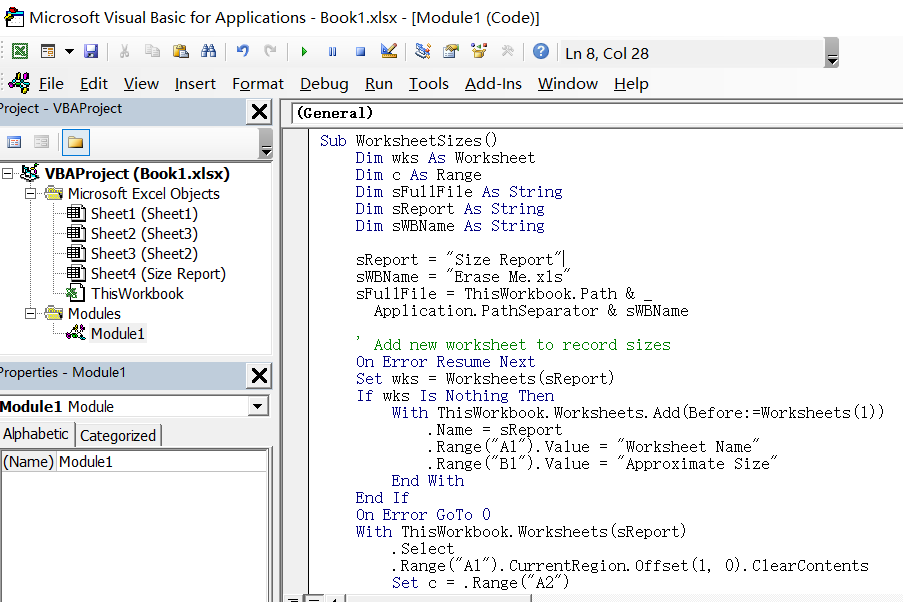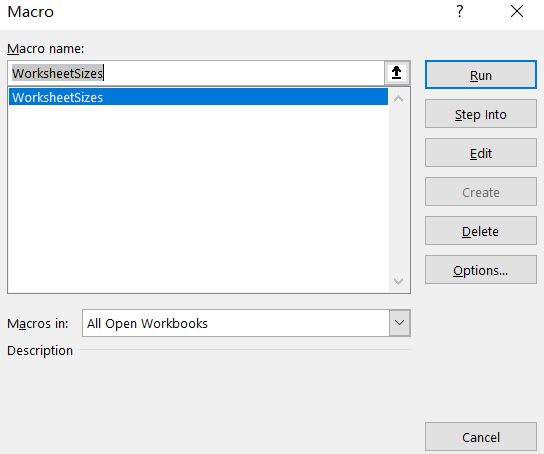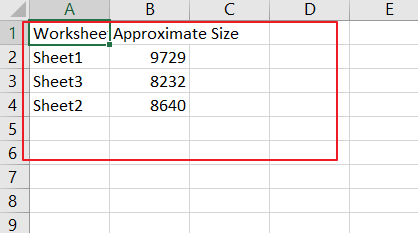# How to Get the Data Size of Each Worksheet in a Workbook

This post will guide you how to check the size of each worksheet of workbook in Excel. How do I get the data size of each worksheet in a workbook in Excel 2013/2016.

Assuming that you have a Workbook which contains multiple worksheet, and you want to know the data size of each worksheet in the current workbook. This post will show two methods with you.

## Method1: Get the Data Size of Each Worksheet by Manually

You can get the data size of each worksheet one by one manually, and you just need to copy the contents of an Excel worksheet in your workbook, and then create a new workbook, and pasted the content into sheet1 in the new workbook, and then save the new workbook to a windows folder. Then you can get the size of this newly workbook.

You can repeat the above steps to get the data sizes of other worksheets one by one in your workbook.

## Method2: Get the Data Size of Each Worksheet via VBA Macro

If you want to quickly get the data size of each worksheet in your current workbook, you can use an Excel VBA macro to accomplish it. Just do the following steps:

Step1: open your excel workbook and then click on “Visual Basic” command under DEVELOPER Tab, or just press “ALT+F11” shortcut.

Step2: then the “Visual Basic Editor” window will appear.

Step3: click “Insert” ->”Module” to create a new module.

Step4: paste the below VBA code  into the code window. Then clicking “Save” button.```Sub WorksheetSizes()
Dim wks As Worksheet
Dim c As Range
Dim sFullFile As String
Dim sReport As String
Dim sWBName As String

sReport = "Size Report"
sWBName = "Erase Me.xls"
sFullFile = ThisWorkbook.Path & _
Application.PathSeparator & sWBName

' Add new worksheet to record sizes
On Error Resume Next
Set wks = Worksheets(sReport)
If wks Is Nothing Then
.Name = sReport
.Range("A1").Value = "Worksheet Name"
.Range("B1").Value = "Approximate Size"
End With
End If
On Error GoTo 0
With ThisWorkbook.Worksheets(sReport)
.Select
.Range("A1").CurrentRegion.Offset(1, 0).ClearContents
Set c = .Range("A2")
End With

Application.ScreenUpdating = False
' Loop through worksheets
For Each wks In ActiveWorkbook.Worksheets
If wks.Name <> sReport Then
wks.Copy
ActiveWorkbook.SaveAs sFullFile
ActiveWorkbook.Close SaveChanges:=False
c.Offset(0, 0).Value = wks.Name
c.Offset(0, 1).Value = FileLen(sFullFile)
Set c = c.Offset(1, 0)
Kill sFullFile
End If
Next wks
Application.ScreenUpdating = True
End Sub

```

Step5: back to the current worksheet, then run the above excel macro. Click Run button.Step6: let’s see the last result:Sidebar